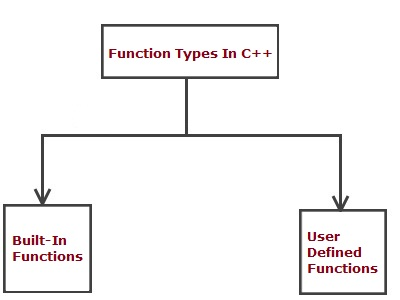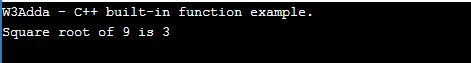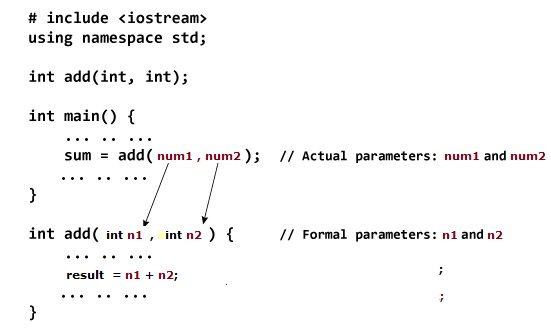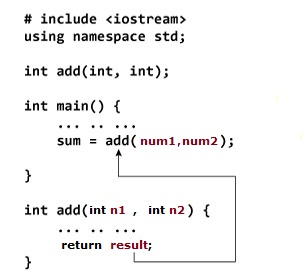C++ Functions

In this tutorial you will learn about the C++ Functions and its application with practical example.

C++ Functions

In C++, functions are one of the key building block of any C++ program. In C++, function gives you way to wrap up the set of statements to perform any specific task and give it a name, so that it can be invoked later from any where in the program. Functions makes it easy to divide the complete program into sub-units that perform a specific task for that program, this way it enhance the modular approach and increase the code re-usability of the program. We pass information in function call as its parameter and the function can either returns some value to the point it where it called from or returns nothing.

• It enhance the modularity of the program.
• It enhance the re usability.
• It makes development easy as development can be shared in team.
• It reduces the coupling.
• It reduces duplication.

Type Of Function

• Built in function
• User defined functionsC++ Built In Function

Built in functions are the functions implicitly available in C++ Programming Language to perform some common and standard tasks that includes the functions for file access, mathematical computations, graphics, memory management etc. Built in function can be accessed simply by including the relative header file using #include directive and at point of function call by just writing the function name, followed by an optional list of arguments.

Built-in functions are also known as library functions. We need not to declare and define these functions as they are already written in the C++ libraries such as iostream, cmath etc. We can directly call them when we need.

Example:-

Here we are using built-in function sqrt(x) that returns the square root of given number x (Double value). This function is declared in cmath header file so we have included the file in our program using #include directive.

Output:-C++ User defined functions

User defined function are custom function defined by user it self to perform as custom task that is not available as built in, in this way user can define and write subprograms as functions to perform a task relevant to their programs. Function gives you way to wrap up the set of statements to perform any specific task and give it a name, so that it can be invoked later from any where in the program.

Function Declaration(Prototype)

A function must be defined prior to use inside main() function, otherwise this will show a compile time error as the main() function is unaware of this user-defined function, its argument list and the return type. In C++, when you define a function before the main() function in your program then it is not required to declare that function but if you are going to provide function definition after the main() function, then it is necessary to provide function prototype by declaring the function first, otherwise this will give compilation error.

Declaring a function In C++

In C++, you can declare a function using “function” keyword as following –

Syntax:-

Above is the general syntax to declare a function prototype, here

function :- It is keyword which is used to define a function.
return_type:- It represents return type of function.
func_name :- It is replaced with the name of the function.
parameter_list :- It represents the list of the parameters need to be passed when function call made.

Example:-

Defining Function

In function definition full body of function is provided. All of the statements that needs to be executed when function is invoked are written here in function body. A function must be defined prior to use, you can define a function as following –

Syntax:-

Example:-

Calling function

In C++, once a function is defined, later you can invoke or call this function in main() function body as following –

Syntax:-

Example:-

When a function is called, the control is transferred to the function body and the statements in function body are executed sequentially. When all of the statement inside function body is executed, the control again moved to the from where the function being invoked with the return value(if any).

Passing Arguments to Function

In C++, when a function is invoked it may be provided with some information as per the function prototype is called as argument (parameter).In the above example, we have two variables num1 and num2 to be passed to add() function when function being called. These arguments are referred to as actual arguments.Then, the variable n1 and n2 are initialized with the value of num1 and num2 respectively. Here, n1 and n2 are referred to as formal arguments.

Return Value from Function

Sometimes we may want a function to return some value to the point it where it is called from. In C++, there is return keyword allows a function to return value.Syntax:-

Example:-

Complete Function In Action

Below is a C++ Program to add two numbers using function.

Example:-

When you run the above c++ program, you will see following output –

Output:-In this tutorial we have learn about the C++ Functions and its application with practical example. I hope you will like this tutorial.